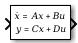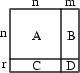# State-Space

Implement linear state-space system

• Library:

•## Description

The State-Space block implements a system whose behavior you define as

`$\begin{array}{l}\stackrel{˙}{x}=Ax+Bu\\ y=Cx+Du\\ {x|}_{t={t}_{0}}={x}_{0},\end{array}$`

where x is the state vector, u is the input vector, y is the output vector, and x0 is the initial condition of the state vector. The matrix coefficients must have these characteristics:

• A must be an n-by-n matrix, where n is the number of states.

• B must be an n-by-m matrix, where m is the number of inputs.

• C must be an r-by-n matrix, where r is the number of outputs.

• D must be an r-by-m matrix.In general, the block has one input port and one output port. The number of rows in C or D matrix is the same as the width of the output port. The number of columns in the B or D matrix are the same as the width of the input port. If you want to model an autonomous linear system with no inputs, set the B and D matrices to empty. In this case, the block acts as a source block with no input port and one output port, and implements the following system:

`$\begin{array}{l}\stackrel{˙}{x}=Ax\\ y=Cx\\ {x|}_{t={t}_{0}}={x}_{0}.\end{array}$`

Simulink® software converts a matrix containing zeros to a sparse matrix for efficient multiplication.

## Ports

### Input

expand all

Real-valued input vector of type `double`, where the width equals the number of columns in the B and D matrices. For more information, see Description.

Data Types: `double`

### Output

expand all

Real-valued output vector of data type `double`, with width equal to the number of rows in the C and D matrices. For more information, see Description.

Data Types: `double`

## Parameters

expand all

Specify the matrix coefficient `A`, as a real-valued n-by-n matrix, where n is the number of states. For more information on the matrix coefficients, see Description.

#### Programmatic Use

 Block Parameter: `A` Type: character vector, string Values: scalar | vector | matrix Default: `'1'`

Specify the matrix coefficient `B`, as a real-valued n-by-m matrix, where n is the number of states and m is the number of inputs. For more information on the matrix coefficients, see Description.

#### Programmatic Use

 Block Parameter: `B` Type: character vector, string Values: scalar | vector | matrix Default: `'1'`

Specify the matrix coefficient C as a real-valued r-by-n matrix, where r is the number of outputs and n is the number of states. For more information on the matrix coefficients, see Description.

#### Programmatic Use

 Block Parameter: `C` Type: character vector, string Values: scalar | vector | matrix Default: `'1'`

Specify the matrix coefficient D as a real-valued r-by-m matrix, where r is the number of outputs and m is the number of inputs. For more information on the matrix coefficients, see Description.

#### Programmatic Use

 Block Parameter: `D` Type: character vector, string Values: scalar | vector | matrix Default: `'1'`

Specify the initial state vector.

#### Limitations

The initial conditions of this block cannot be `inf` or `NaN`.

#### Programmatic Use

 Block Parameter: `X0` Type: character vector, string Values: scalar | vector Default: `'0'`

Absolute tolerance for computing block states, specified as a positive, real-valued, scalar or vector. To inherit the absolute tolerance from the Configuration Parameters, specify `auto` or `-1`.

• If you enter a real scalar, then that value overrides the absolute tolerance in the Configuration Parameters dialog box for computing all block states.

• If you enter a real vector, then the dimension of that vector must match the dimension of the continuous states in the block. These values override the absolute tolerance in the Configuration Parameters dialog box.

• If you enter `auto` or –1, then Simulink uses the absolute tolerance value in the Configuration Parameters dialog box (see Solver Pane) to compute block states.

#### Programmatic Use

 Block Parameter: ` AbsoluteTolerance` Type: character vector, string Values: `'auto'` | `'-1'` | any positive real-valued scalar or vector Default: `'auto'`

Assign a unique name to each state. If this field is blank (`' '`), no name assignment occurs.

• To assign a name to a single state, enter the name between quotes, for example, `'position'`.

• To assign names to multiple states, enter a comma-delimited list surrounded by braces, for example, `{'a', 'b', 'c'}`. Each name must be unique.

• To assign state names with a variable in the MATLAB® workspace, enter the variable without quotes. A variable can be a character vector, string, cell array, or structure.

#### Limitations

• The state names apply only to the selected block.

• The number of states must divide evenly among the number of state names.

• You can specify fewer names than states, but you cannot specify more names than states.

For example, you can specify two names in a system with four states. The first name applies to the first two states and the second name to the last two states.

#### Programmatic Use

 Block Parameter: `ContinuousStateAttributes` Type: character vector, string Values: `' '` | user-defined Default: `' '`

## Block Characteristics

 Data Types `double` Direct Feedthrough `yes` Multidimensional Signals `no` Variable-Size Signals `no` Zero-Crossing Detection `no`